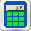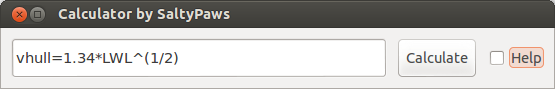## Calculator

This plugin is available for Linux and Windows.

#### Goals

This is a light weight yet powerful calculator plugin for OpenCPN which includes functions to solve nautical questions.

• Would you like to know your hull speed?

• Increase of draft due to heel.

• More functions can be added by the author.

Key features are:

• Storing results in variables

• Shows historic calculations

• Full complement of nautical functions

• Screen footprint can be optimized & minimized as required

#### Requirements

``````OpenCPN 4.2.0 and above.
Linux or Windows``````

### Use

The calculator plugin allows you to carry out all nautical calculations, without having to leave the OpenCPN environment. The scientific calculator is capable of working with, and retaining variables.
* When starting the plugin for the first time, go toto find the plugin tab. Click on the calculator plugin, then the “Enable” button.

• Back in the OpenCPN menu the calculator iconbrings up the calculator.

•• Tick the “Help” tickbox to bring up a comprehensive help screen. Unticking the help button brings you back to the calculator only interface and resizes the menu to the original size.

• Enter calculations in the box and press enter or “Calculate” for evaluation. Press the up or down button, to retrieve historic input and results (max 30).

#### Help

Type help in the calculator to get these instructions.

#### Examples

Examples of expressions that work in the calculator are: (comments are in brackets, some results depend on other example calculations):

##### Hull speed:
• LWL=48 (water line length in feet)

• vhull=1.34*LWL^(1/2) (hull speed in knots)

##### Conversions:
• ftm=0.3048 (feet to meters)

• km_to_nm=0.539957 (Kilometers to nautical Mile)

• ftm*LWL (waterline length in meters)

##### Distance to horizon
• R=6378.1*1000 (Radius of the earth in m)

• H=2.5 (Height of the eye above sea-level in m)

• d = R * acos(R/(R + h)) (Distance to horizon in m)

• ans*km_to_nm (Distance to horizon in nm)

##### Distance to lighthouse
• H1=200 (height of lighthouse in m)

• d1 = R*acos(R/(R + H1)) (Distance to horizon in m)

• distance=d1+d (visibility range of lighthouse in m)

##### Useful Notes
• Ans is the result of the previous calculation

• Variables can be defined (e.g. myvariable=10/8*cos(dtr*90) or yourvariable=Ans)

• % modulus - Divides the value of one expression by the value of another, and returns the remainder.

• ! factorial

• Sign Returns .-1 for negative numbers and 1 for positive numbers

• Factorial Variables: Pi, e

• dtr is the conversion factor from degrees to radians

#### Built-in functions

The following table gives an overview of the functions supported by the default implementation. It lists the function names, the number of arguments and a brief description.

[.np_break]# #

Name Argc. Explanation

TRIGONOMETRY

default entry use radians e.g. sin(dtr*90) to calculate in degrees

sin

1

sine function

cos

1

cosine function

tan

1

tangens function

asin

1

arcus sine function

acos

1

arcus cosine function

atan

1

arcus tangens function

sinh

1

hyperbolic sine function

cosh

1

hyperbolic cosine

tanh

1

hyperbolic tangens function

asinh

1

hyperbolic arcus sine function

acosh

1

hyperbolic arcus tangens function

atanh

1

hyperbolic arcur tangens function

LOGARITHMIC

log2

1

logarithm to the base 2

log10

1

logarithm to the base 10

log

1

logarithm to the base 10

ln

1

logarithm to base e (2.71828…)

OTHER

exp

1

e raised to the power of x

sqrt

1

square root of a value

sign

1

sign function -1 if x<0; 1 if x>0

rint

1

round to nearest integer

abs

1

absolute value

min

var.

min of all arguments

max

var.

max of all arguments

sum

var.

sum of all arguments

avg

var.

mean value of all arguments

[.np_break]# #

#### Built-in binary operators

The following table lists the default binary operators supported by the parser.

[.np_break]# #

 Operator Meaning Priority = assignment -1 && logical and 1 logical or 2 ⇐ less or equal 4 >= greater or equal 4 != not equal 4 == equal 4 > greater than 4 < less than 4 + addition 5 - subtraction 5 * multiplication 6 / division 6 ^ raise x to the power of y 7 ^

[.np_break]# #

• The assignment operator is special since it changes one of its arguments and can only by applied to variables.

#### Other operators

##### MuParser

muParser has built in support for the if.. then.. else operator. It uses lazy evaluation in order to make sure only the necessary branch of the expression is evaluated.

[.np_break]# #

 Operator Meaning ?: if then else operator

[.np_break]# #

##### Variables:

[.np_break]# #

pi, e well known

ans

the result of the previous calculation

dtr

conversion factor from degrees to radians

Define variables

e.g. myvariable=10/8*cos(dtr*90) or yourvariable=ans

clear

removes results in the history and leaves defined variables in tact

[.np_break]# #

##### User Interface:

Type these commands in the command window:

[.np_break]# #

 history Toggle the history panel showhelp Show/Hide the Help button showcalculate Show/Hide the Calculate button showhistory Show/Hide the history toggle help show the help menu

[.np_break]# #

#### Settings/Plugins/Preferences:

• Show/Hide Calculate

• Help and History toggle buttons

• History Settings: max Results -this is the number of results that will be stored in the history pull down. The history pulldown will contain five times this value.

• Log to opencpn: Enable/Disable logging of results to opencpn logfile.

#### Other:

• Error handling supported

• MuParser has been compiled with C++ Double for internal precision.

#### Compiling

You have to be able to compile OpenCPN itself - Get the info athttp://opencpn.org/ocpn/developers_manual[Devolpers Manual]

Build out of OpenCPN branch!

in folder calculator_pi

• mkdir build

• cd build

• cmake ..

• make

• sudo make installf

After using wxformbuilder to modify the interface, use degreefix.sh, to facilitate the windows build. When updating the Excel file with formulas and conversions, convert to cpp code by running the supplied perl script

#### Changes to version 1.6

• Added wind speed to Beaufort equation

• Changed to MuParser math interface

• Added History pulldown box, improved history box behaviour

• Added reporting modes (1000 separator, SI prefixes, accuracy)

• Added function button in OpenCPN toolbar, with option to switch off from plugin settings

• Minor tweaks and fixes

SaltyPaws aka Walbert Schulpen

#### Support for Diagrams

I believe the plugin supports small jpg & png images for the purpose of better visualization. What is needed to implement this system is some diagrams and more knowledge about the plugin. Rgleason